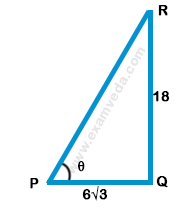# Find the angle of elevation of the sun when the shadow of a pole of 18 m height is $$6\sqrt 3$$ m long?

A. 30°

B. 60°

C. 45°

D. None of these

### Solution(By Examveda Team)Let RQ be the pole and PQ be the shadow
Given that RQ = 18 m and PQ = $$6\sqrt 3$$ m
Let the angle of elevation, ∠RPQ = $$\theta$$
\eqalign{ & {\text{From the right}}\Delta PQR, \cr & {\text{tan}}\,\theta = \frac{{RQ}}{{PQ}} \cr & \,\,\,\,\,\,\,\,\,\,\,\,\,\,\, = \frac{{18}}{{6\sqrt 3 }} \cr & \,\,\,\,\,\,\,\,\,\,\,\,\,\,\, = \frac{3}{{\sqrt 3 }} \cr & \,\,\,\,\,\,\,\,\,\,\,\,\,\,\, = \sqrt 3 \cr & \Rightarrow \theta = {\tan ^{ - 1}}\left( {\sqrt 3 } \right) = {60^ \circ } \cr}

This Question Belongs to Arithmetic Ability >> Height And Distance

Related Questions on Height and Distance